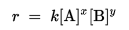# Rate law and specific rate constant

The rate law or rate equation for a chemical reaction is an equation that links the reaction rate with concentrations or pressures of reactants and constant parameters (normally rate coefficients and partial reaction orders). For many reactions the rate is given by a power law such as :where [A] and [B] express the concentration of the species A and B, respectively (usually in moles per liter (molarity, M)). The exponents x and y are the partial reaction ordersand must be determined experimentally; they are often not equal to the stoichiometric coefficients. The constant k is the rate coefficient or rate constant of the reaction.

### Determining Exponents for a Rate Law from Initial Rates (Experimental Data)

If we are given data from two or more experiments at the same temperature with different concentrations of reactants and different rates we can determine the exponents in the differential rate law for the reaction as follows:

1.Write the rate law with the concentrations of all species for which data is given. Write the coefficients as unknowns: n, m, etc. For example, we might have an equation such as the following

rate = k[A]n[B]m

ratios of the experimental data that give different rates

rate1 / rate2 = k[A]n1[B]m1 / k[A]n2[B]m2